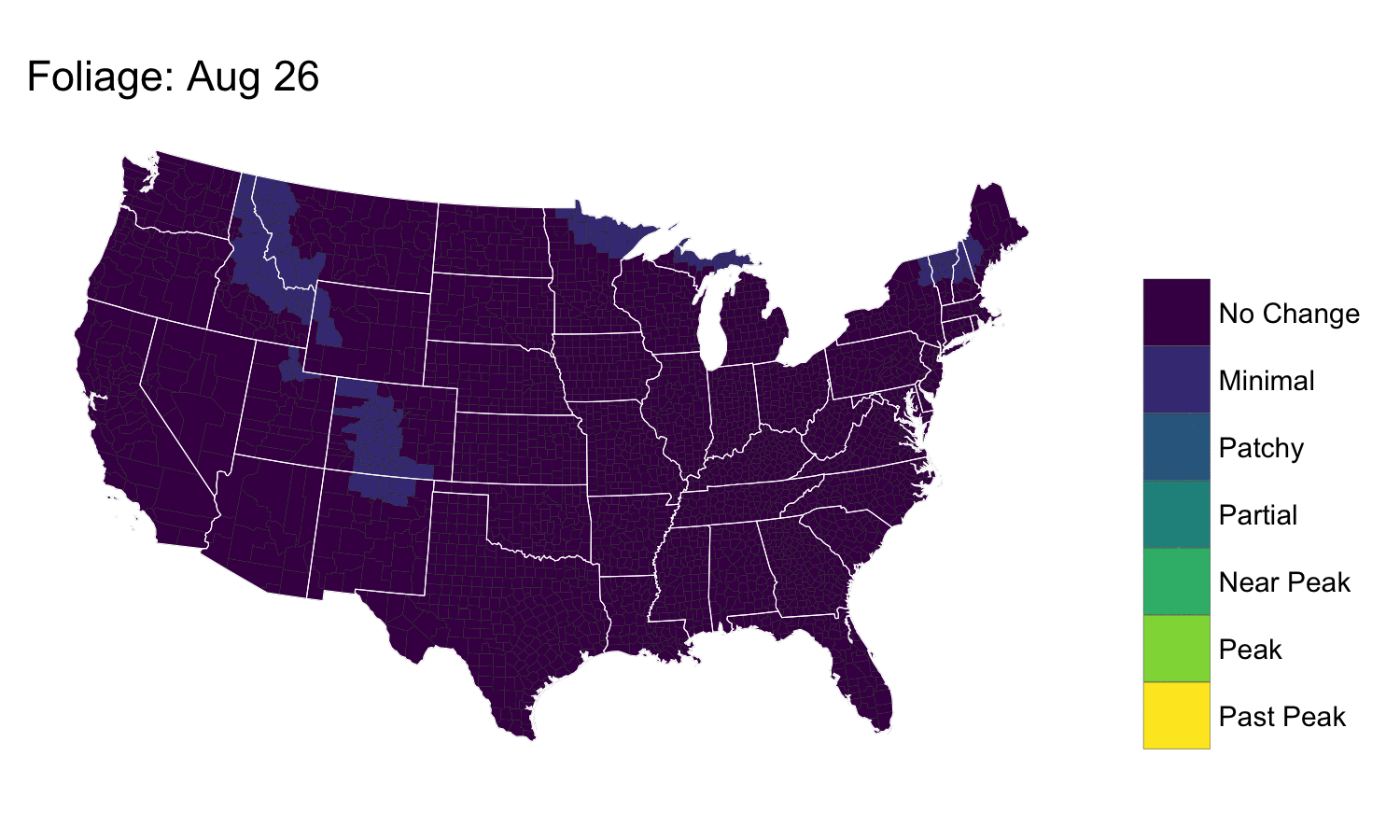Want to share your content on R-bloggers? click here if you have a blog, or here if you don't.

I was socially engineered by @yoniceedee into creating today’s post due to being prodded with this tweet:

Since there aren’t nearly enough `sf` and `geom_sf` examples out on the wild, wild #rstats web, here’s a short one that shows how to do basic `sf` operations, including how to plot `sf` objects in ggplot2 and animate a series of them with `magick`.

I’m hoping someone riffs off of this to make an interactive version with Shiny. If you do, definitely drop a link+note in the comments!

(If folks want some exposition here, let me know in the comments and I’ll rig up an R Markdown document with more step-by-step details.)

Full RStudio project file (with pre-cached data) is on GitHub.```library(rprojroot)
library(sf)
library(magick)
library(tidyverse) # NOTE: Needs github version of ggplot2

root <- find_rstudio_root_file()

# "borrow" the files from SmokyMountains.com, but be nice and cache them to
# avoid hitting their web server for every iteration

c("https://smokymountains.com/wp-content/themes/smcom-2015/to-delete/js/us.json",
"https://smokymountains.com/wp-content/themes/smcom-2015/js/foliage2.tsv",
"https://smokymountains.com/wp-content/themes/smcom-2015/js/foliage-2017.csv") %>%
walk(~{
sav_tmp <- file.path(root, "data", basename(.x))
})

# next, we read in the GeoJSON file twice. first, to get the counties
states_sf <- read_sf(file.path(root, "data", "us.json"), "states", stringsAsFactors = FALSE)

# we only want the continental US
states_sf <- filter(states_sf, !(id %in% c("2", "15", "72", "78")))

# it doesn't have a CRS so we give it one
st_crs(states_sf) <- 4326

# I ran into hiccups using coord_sf() to do this, so we convert it to Albers here
states_sf <- st_transform(states_sf, 5070)

# next we read in the states
counties_sf <- read_sf(file.path(root, "data", "us.json"), "counties", stringsAsFactors = FALSE)
st_crs(counties_sf) <- 4326
counties_sf <- st_transform(counties_sf, 5070)

# now, we read in the foliage data
col_types = cols(.default=col_double(), id=col_character()))

# and, since we have a lovely sf tidy data frame, bind it together
left_join(counties_sf, foliage, "id") %>%
filter(!is.na(rate1)) -> foliage_sf

# now, we do some munging so we have better labels and so we can
# iterate over the weeks
gather(foliage_sf, week, value, -id, -geometry) %>%
mutate(value = factor(value)) %>%
filter(week != "rate1") %>%
mutate(week = factor(week,
levels=unique(week),
labels=format(seq(as.Date("2017-08-26"),
as.Date("2017-11-11"), "1 week"),
"%b %d"))) -> foliage_sf

# now we make a ggplot object for each week and save it out to a png
pb <- progress_estimated(nlevels(foliage_sf\$week))
walk(1:nlevels(foliage_sf\$week), ~{

pb\$tick()\$print()

xdf <- filter(foliage_sf, week == levels(week)[.x])

ggplot() +
geom_sf(data=xdf, aes(fill=value), size=0.05, color="#2b2b2b") +
geom_sf(data=states_sf, color="white", size=0.125, fill=NA) +
viridis::scale_fill_viridis(
name=NULL,
discrete = TRUE,
labels=c("No Change", "Minimal", "Patchy", "Partial", "Near Peak", "Peak", "Past Peak"),
drop=FALSE
) +
labs(title=sprintf("Foliage: %s ", unique(xdf\$week))) +
ggthemes::theme_map() +
theme(panel.grid=element_line(color="#00000000")) +
theme(panel.grid.major=element_line(color="#00000000")) +
theme(legend.position="right") -> gg

ggsave(sprintf("%02d.png", .x), gg, width=5, height=3)

})

# we read them all back in and animate the foliage
sprintf("%02d.png", 1:nlevels(foliage_sf\$week)) %>%